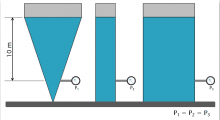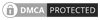## Hydrostatics and hydraulic calculationsHydrostatics is a branch of hydraulics that deals with fluids at rest. As mentioned earlier, water is the single most important component of fire protection and hydraulic calculations, so in this section will look at water at rest.

### Basic Principles

1. The pressure at a point in the liquid is equal in every direction.
2. Pressure applied from an external source on a confined liquid will be transmitted in all directions throughout the liquid without a reduction in magnitude.
3. The pressure created by a liquid in an open container is directly proportional to the depth of the liquid.
4. The pressure created by a liquid in an open container is directly proportional to the density of the liquid.
5. The shape or volume of a liquid has no influence on the pressure created by the liquid.

A common misunderstanding is that pressure always acts in a download direction, this fallacy is fostered by the understanding that pressure is related to weight. Principle 1 which is known as Pascal's Law points out that pressure acts in all directions as shown in figure 1. The four pressure gauges are in different orientations but taking a pressure reading from the same point, all four gauges will show the same pressure.Principle two has obvious practical limitations. The idea of undiminished pressure transmission throughout a confined liquid would be more readily accepted if the incompressible nature of liquids is more fully understood. Gases will contract in volume when placed under pressure, whereas liquids will maintain their volume when placed under pressure. Because the volume is not reduced, the increase in pressure is propagated throughout the entirety of the liquid in every direction with no drop in magnitude.

Principle three is an essential part of fire reduction hydraulics. The pressure created by a column of liquid is directly proportional to the depth of the liquid. Water has a density of 1000kg/m3. Therefore, 1 cubic metre of water will weigh 1000kg.

Principle four is closely linked to principle three in that pressure created by a liquid is also directly proportional to the density. Mercury, for example, has a density that is 13.6 times greater than water, so the pressure created by 1 metre of mercury will be 13.6 times greater than the pressure created by 1 metre of water. Both principle three and four can be summarized by the following relationship:

P=w.h

Where:

• P = Pressure (bar)
• w = specific weight of the liquid in kg/m3.
• h = height of the liquid column in metres.

Principle five is generally the most difficult to understand. Pressure developed is not related to size or shape of the container, only the depth and density of the liquid. Principle five applies to pressure, and not to force. A column of water has the same pressure throughout but will have different weights depending on where the pressure reading is taken. Pressure only depends on the depth of the liquid, and is independent of the shape or volume of the container.It is common to hear fire protection specialists refer to water pressure in terms of head. Head is pressure expressed in units of metres of water rather than bar, and can be expressed in the general form:

h= P/w

Where:

• h = head in metres.
• P = pressure (bar).
• w = the specific weight in kg/m3.

### Buoyancy

Buoyancy is where an object floats on the surface of a liquid. For an object to do this, the force in the downwards direction must be equalised by a force in the upward direction. The force acting downwards will equal the weight density of the material times the volume of the object.

F1=w.V

In order for the object to remain stationary, F1, must also equal w.V. The amount of material that sticks above the surface of the liquid, and if an object will float, depends upon the relationship between the weight densities of the objects and the liquid. A solid object will float in a liquid only if its weight density is less than or equal to that of the liquid. The proportion of a floating object that will sink below the surface of a liquid will depend upon how much of the liquid will equal the weight of the object.

### Potential Energy

It is important to understand the part that hydrostatic pressure plays in the energy composition of a hydraulic system. Hydrostatic pressure composes the potential energy component of the total energy of a hydraulic system. Potential energy is stored energy. It has the ability to perform work once released. It is easy to visualise the potential of a stretched rubber band or a coiled spring. However, elevated or pressurised water also contains potential energy. Because energy is the ability to do work, and work is the product of force times distance and has units of metre-kilograms; potential energy is the potential or ability to move some unit of weight (force) some distance, or:

PE=W.h

Where:

• W = weight in kilograms.
• h = height in metres.Canute LLP
15 Queen Square
Leeds, West Yorkshire
United Kingdom LS2 8AJ

t: +44 (0) 113 328 0350
e: info@canutesoft.com

 Copyright © 2021, Canute LLPRegistered in England & Wales, Partnership No. OC305985